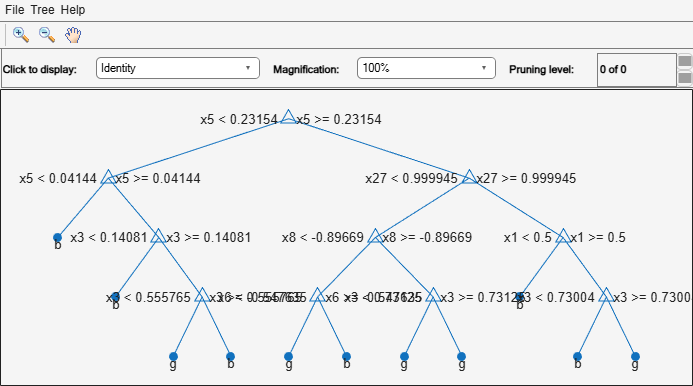# CompactClassificationEnsemble

Package: classreg.learning.classif

Compact classification ensemble class

## Description

Compact version of a classification ensemble (of class `ClassificationEnsemble`). The compact version does not include the data for training the classification ensemble. Therefore, you cannot perform some tasks with a compact classification ensemble, such as cross validation. Use a compact classification ensemble for making predictions (classifications) of new data.

## Construction

```ens = compact(fullEns)``` constructs a compact decision ensemble from a full decision ensemble.

### Input Arguments

 `fullEns` A classification ensemble created by `fitcensemble`.

## Properties

 `CategoricalPredictors` Categorical predictor indices, specified as a vector of positive integers. `CategoricalPredictors` contains index values indicating that the corresponding predictors are categorical. The index values are between 1 and `p`, where `p` is the number of predictors used to train the model. If none of the predictors are categorical, then this property is empty (`[]`). `ClassNames` List of the elements in `Y` with duplicates removed. `ClassNames` can be a numeric vector, vector of categorical variables, logical vector, character array, or cell array of character vectors. `ClassNames` has the same data type as the data in the argument `Y`. (The software treats string arrays as cell arrays of character vectors.) `CombineWeights` Character vector describing how `ens` combines weak learner weights, either `'WeightedSum'` or `'WeightedAverage'`. `Cost` Square matrix, where `Cost(i,j)` is the cost of classifying a point into class `j` if its true class is `i` (the rows correspond to the true class and the columns correspond to the predicted class). The order of the rows and columns of `Cost` corresponds to the order of the classes in `ClassNames`. The number of rows and columns in `Cost` is the number of unique classes in the response. This property is read-only. `ExpandedPredictorNames` Expanded predictor names, stored as a cell array of character vectors. If the model uses encoding for categorical variables, then `ExpandedPredictorNames` includes the names that describe the expanded variables. Otherwise, `ExpandedPredictorNames` is the same as `PredictorNames`. `NumTrained` Number of trained weak learners in `ens`, a scalar. `PredictorNames ` A cell array of names for the predictor variables, in the order in which they appear in `X`. `Prior` Numeric vector of prior probabilities for each class. The order of the elements of `Prior` corresponds to the order of the classes in `ClassNames`. The number of elements of `Prior` is the number of unique classes in the response. This property is read-only. `ResponseName` Character vector with the name of the response variable `Y`. `ScoreTransform` Function handle for transforming scores, or character vector representing a built-in transformation function. `'none'` means no transformation; equivalently, `'none'` means `@(x)x`. For a list of built-in transformation functions and the syntax of custom transformation functions, see `fitctree`. Add or change a `ScoreTransform` function using dot notation: `ens.ScoreTransform = 'function'` or `ens.ScoreTransform = @function` `Trained` A cell vector of trained classification models. If `Method` is `'LogitBoost'` or `'GentleBoost'`, then `CompactClassificationEnsemble` stores trained learner `j` in the `CompactRegressionLearner` property of the object stored in `Trained{j}`. That is, to access trained learner `j`, use `ens.Trained{j}.CompactRegressionLearner`.Otherwise, cells of the cell vector contain the corresponding, compact classification models. `TrainedWeights` Numeric vector of trained weights for the weak learners in `ens`. `TrainedWeights` has `T` elements, where `T` is the number of weak learners in `learners`. `UsePredForLearner` Logical matrix of size `P`-by-`NumTrained`, where `P` is the number of predictors (columns) in the training data `X`. `UsePredForLearner(i,j)` is `true` when learner `j` uses predictor `i`, and is `false` otherwise. For each learner, the predictors have the same order as the columns in the training data `X`. If the ensemble is not of type `Subspace`, all entries in `UsePredForLearner` are `true`.

## Object Functions

 `compareHoldout` Compare accuracies of two classification models using new data `edge` Classification edge `gather` Gather properties of Statistics and Machine Learning Toolbox object from GPU `lime` Local interpretable model-agnostic explanations (LIME) `loss` Classification error `margin` Classification margins `partialDependence` Compute partial dependence `plotPartialDependence` Create partial dependence plot (PDP) and individual conditional expectation (ICE) plots `predict` Classify observations using ensemble of classification models `predictorImportance` Estimates of predictor importance for classification ensemble of decision trees `removeLearners` Remove members of compact classification ensemble `shapley` Shapley values

## Copy Semantics

Value. To learn how value classes affect copy operations, see Copying Objects.

## Examples

collapse all

Create a compact classification ensemble for efficiently making predictions on new data.

Load the `ionosphere` data set.

`load ionosphere`

Train a boosted ensemble of 100 classification trees using all measurements and the `AdaBoostM1` method.

`Mdl = fitcensemble(X,Y,'Method','AdaBoostM1')`
```Mdl = ClassificationEnsemble ResponseName: 'Y' CategoricalPredictors: [] ClassNames: {'b' 'g'} ScoreTransform: 'none' NumObservations: 351 NumTrained: 100 Method: 'AdaBoostM1' LearnerNames: {'Tree'} ReasonForTermination: 'Terminated normally after completing the requested number of training cycles.' FitInfo: [100x1 double] FitInfoDescription: {2x1 cell} Properties, Methods ```

`Mdl` is a `ClassificationEnsemble` model object that contains the training data, among other things.

Create a compact version of `Mdl`.

`CMdl = compact(Mdl)`
```CMdl = CompactClassificationEnsemble ResponseName: 'Y' CategoricalPredictors: [] ClassNames: {'b' 'g'} ScoreTransform: 'none' NumTrained: 100 Properties, Methods ```

`CMdl` is a `CompactClassificationEnsemble` model object. `CMdl` is almost the same as `Mdl`. One exception is that `CMdl` does not store the training data.

Compare the amounts of space consumed by `Mdl` and `CMdl`.

```mdlInfo = whos('Mdl'); cMdlInfo = whos('CMdl'); [mdlInfo.bytes cMdlInfo.bytes]```
```ans = 1×2 878354 631686 ```

`Mdl` consumes more space than `CMdl`.

`CMdl.Trained` stores the trained classification trees (`CompactClassificationTree` model objects) that compose `Mdl`.

Display a graph of the first tree in the compact ensemble.

`view(CMdl.Trained{1},'Mode','graph');`By default, `fitcensemble` grows shallow trees for boosted ensembles of trees.

Predict the label of the mean of `X` using the compact ensemble.

`predMeanX = predict(CMdl,mean(X))`
```predMeanX = 1x1 cell array {'g'} ```

## Tips

For an ensemble of classification trees, the `Trained` property of `ens` stores an `ens.NumTrained`-by-1 cell vector of compact classification models. For a textual or graphical display of tree `t` in the cell vector, enter:

• `view(ens.Trained{t}.CompactRegressionLearner)` for ensembles aggregated using LogitBoost or GentleBoost.

• `view(ens.Trained{t})` for all other aggregation methods.

## Version History

Introduced in R2011a

expand all